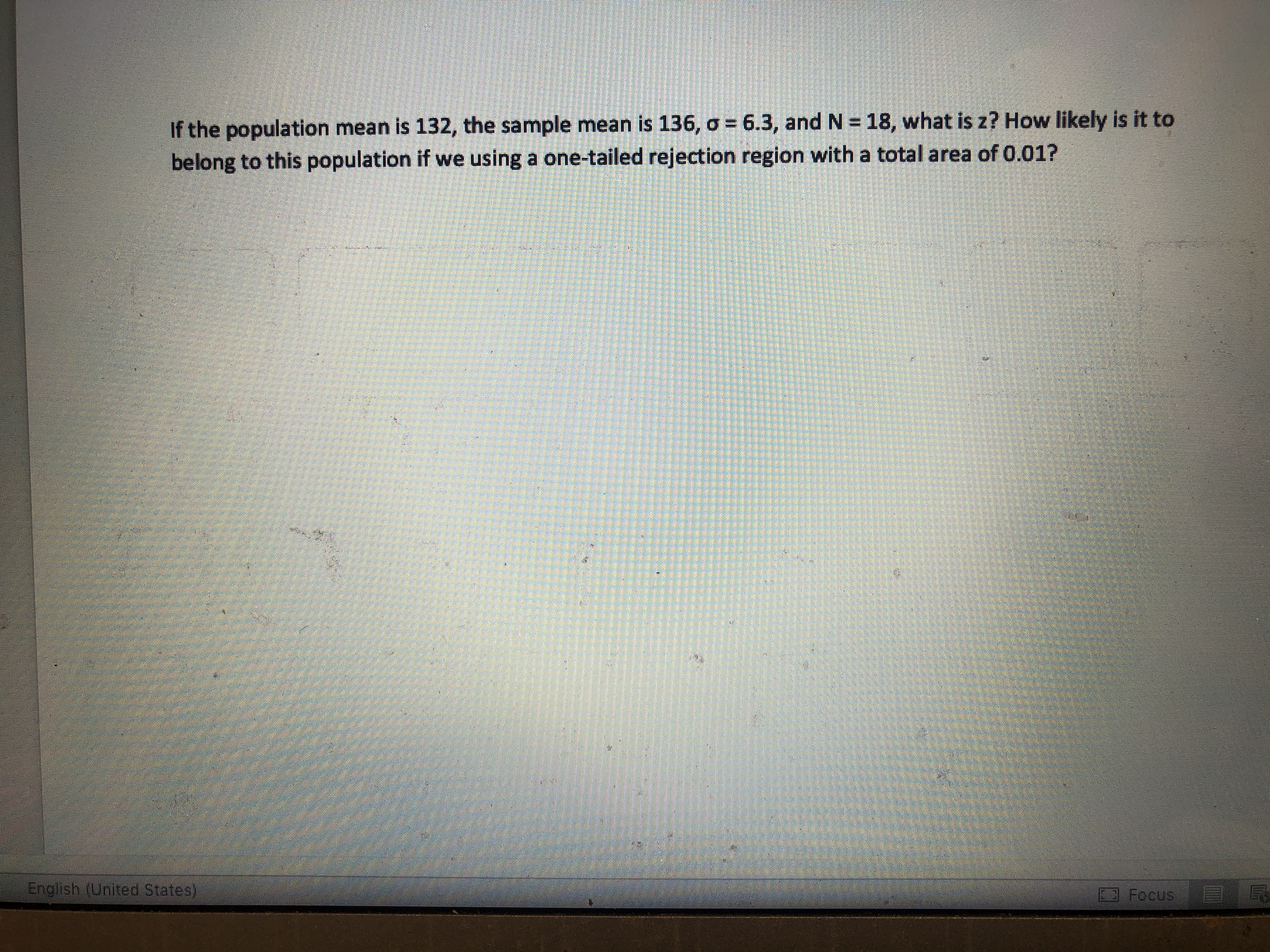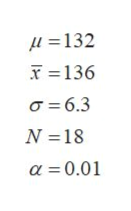If the population mean is 132, the sample mean is 136, o 6.3, and N 18, what is z? How likely is it tobelong to this population if we using a one-tailed rejection region with a total area of 0.01?English (United States)Focus

Questionhelp_outlineImage TranscriptioncloseIf the population mean is 132, the sample mean is 136, o 6.3, and N 18, what is z? How likely is it to belong to this population if we using a one-tailed rejection region with a total area of 0.01? English (United States) Focus fullscreen
Step 1

The provided information is,help_outlineImage TranscriptioncloseL=132 T 136 a 6.3 N=18 a 0.01 fullscreen
Step 2

The z-value can be obtained as:

Step 3

The hypotheses can b...

Want to see the full answer?

See Solution

Want to see this answer and more?

Our solutions are written by experts, many with advanced degrees, and available 24/7

See Solution
Tagged in

Statistics